Publicité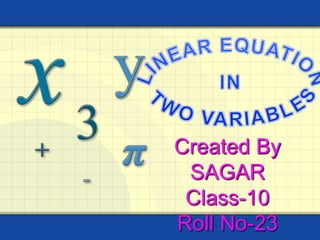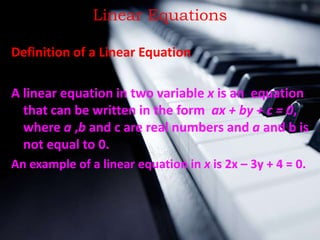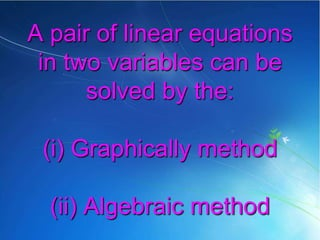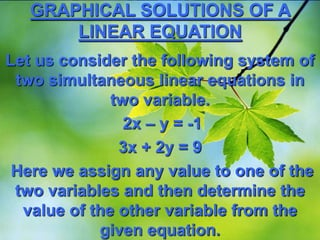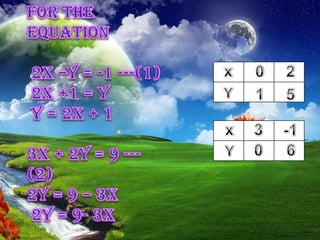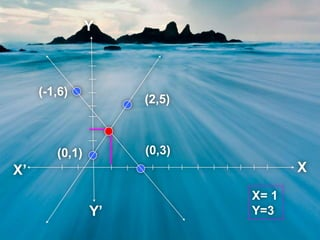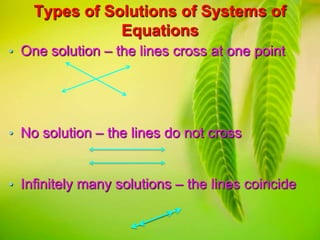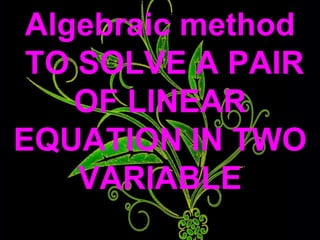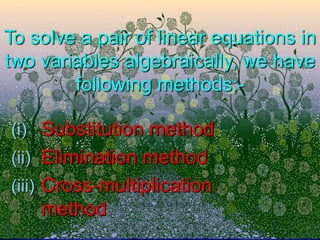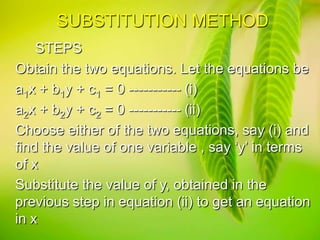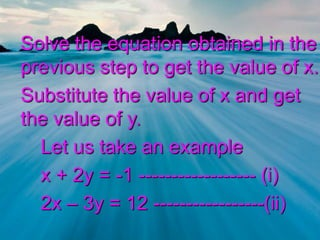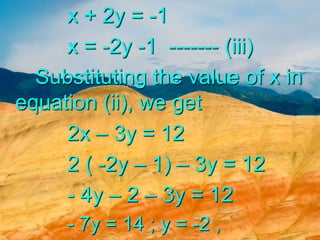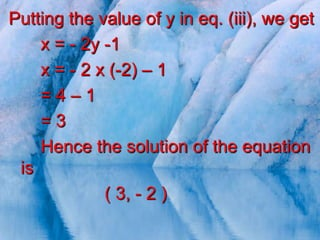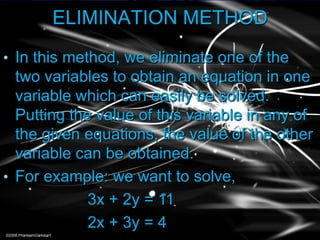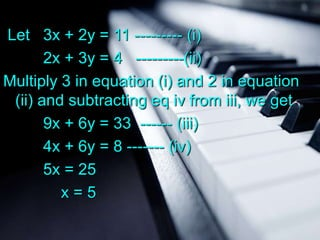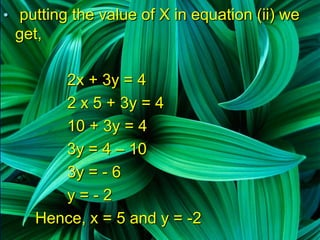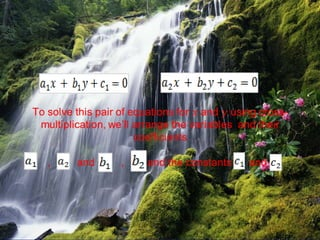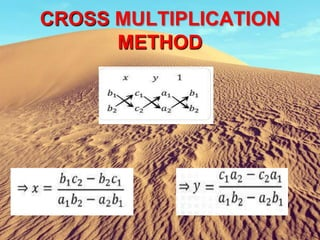1 sur 19
Publicité

### PPT on Linear Equations in two variables

1. Created By SAGAR Class-10 Roll No-23
2. Linear Equations Definition of a Linear Equation A linear equation in two variable x is an equation that can be written in the form ax + by + c = 0, where a ,b and c are real numbers and a and b is not equal to 0. An example of a linear equation in x is 2x – 3y + 4 = 0.
3. A pair of linear equations in two variables can be solved by the: (i) Graphically method (ii) Algebraic method
4. GRAPHICAL SOLUTIONS OF A LINEAR EQUATION Let us consider the following system of two simultaneous linear equations in two variable. 2x – y = -1 3x + 2y = 9 Here we assign any value to one of the two variables and then determine the value of the other variable from the given equation.
5. xxx 00
6. Types of Solutions of Systems of Equations • One solution – the lines cross at one point • No solution – the lines do not cross • Infinitely many solutions – the lines coincide
7. Algebraic method TO SOLVE A PAIR OF LINEAR EQUATION IN TWO VARIABLE
8. To solve a pair of linear equations in two variables algebraically, we have following methods:(i) Substitution method (ii) Elimination method (iii) Cross-multiplication method
9. SUBSTITUTION METHOD STEPS Obtain the two equations. Let the equations be a1x + b1y + c1 = 0 ----------- (i) a2x + b2y + c2 = 0 ----------- (ii) Choose either of the two equations, say (i) and find the value of one variable , say ‘y’ in terms of x Substitute the value of y, obtained in the previous step in equation (ii) to get an equation in x
10. Solve the equation obtained in the previous step to get the value of x. Substitute the value of x and get the value of y. Let us take an example x + 2y = -1 ------------------ (i) 2x – 3y = 12 -----------------(ii)
11. x + 2y = -1 x = -2y -1 ------- (iii) Substituting the value of x in equation (ii), we get 2x – 3y = 12 2 ( -2y – 1) – 3y = 12 - 4y – 2 – 3y = 12 - 7y = 14 ; y = -2 ,
12. Putting the value of y in eq. (iii), we get x = - 2y -1 x = - 2 x (-2) – 1 =4–1 =3 Hence the solution of the equation is ( 3, - 2 )
13. ELIMINATION METHOD • In this method, we eliminate one of the two variables to obtain an equation in one variable which can easily be solved. Putting the value of this variable in any of the given equations, the value of the other variable can be obtained. • For example: we want to solve, 3x + 2y = 11 2x + 3y = 4
14. Let 3x + 2y = 11 --------- (i) 2x + 3y = 4 ---------(ii) Multiply 3 in equation (i) and 2 in equation (ii) and subtracting eq iv from iii, we get 9x + 6y = 33 ------ (iii) 4x + 6y = 8 ------- (iv) 5x = 25 x=5
15. • putting the value of X in equation (ii) we get, 2x + 3y = 4 2 x 5 + 3y = 4 10 + 3y = 4 3y = 4 – 10 3y = - 6 y=-2 Hence, x = 5 and y = -2
16. CROSS MULTIPLICATION METHOD
Publicité